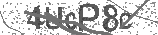﻿ The Adding by 3 Song (Math Facts) - Addition Song for Kids | Silly School Songs | Safe Videos for Kids
Welcome

# The Adding by 3 Song (Math Facts) - Addition Song for Kids | Silly School Songs

Thanks! Share it with your friends!

URL

You disliked this video. Thanks for the feedback!

Sorry, only registred users can create playlists.URLChannel: Silly School Songs
Categories: Basic Math   |   MathFind Related Videos  added
148 Views## Description

Learn how to add by 3 with this fun and memorable song that will have you tapping your feet! For more music and information, visit http://sillyschoolsongs.com.

Lyrics:

Alright kids, are you ready to learn how to add by 3?
"Yeah!"
Well, let's go ahead and do it!
Here we go!
3 + 0 = 3
3 + 1 = 4
3 + 2 = 5
3 + 3 = 6
3 + 4 = 7
3 + 5 = 8
3 + 6 = 9
3 + 7 = 10
3 + 8 = 11
3 + 9 = 12
3 + 10 = 13
It's pretty simple...you know what I mean?
Excellent job!
Alright, it's time for us to turn the numbers around,
Start with 0 + 3 and go up.
Ready?
Here we go!
0 + 3 = 3
1 + 3 = 4
2 + 3 = 5
3 + 3 = 6
4 + 3 = 7
5 + 3 = 8
6 + 3 = 9
7 + 3 = 10
8 + 3 = 11
9 + 3 = 12
10 + 3 = 13
Well, that was simple, right?
Now we're going to go all the way up,
and then all the way back down.
Ready?
Here we go!
3 + 0 = 3
3 + 1 = 4
3 + 2 = 5
3 + 3 = 6
3 + 4 = 7
3 + 5 = 8
3 + 6 = 9
3 + 7 = 10
3 + 8 = 11
3 + 9 = 12
3 + 10 = 13
Now it's time to go back down
10 + 3 = 13
9 + 3 = 12
8 + 3 = 11
7 + 3 = 10
6 + 3 = 9
5 + 3 = 8
4 + 3 = 7
3 + 3 = 6
2 + 3 = 5
1 + 3 = 4
0 + 3 = 3
That's it!

Copyright 2015 Silly School Songs. All Rights Reserved.## Post your comment## Comments

Be the first to comment